Question

# Calculate the position of the center of mass of CO, measured from the O atom, which...

Calculate the position of the center of mass of CO, measured from the O atom, which has a bond length of 112.8 pm. The isotopic mass of 12С atom is 12.0000 amu and the isotopic mass of O atom is 15.9949 amu

Please work out without using graph, if possible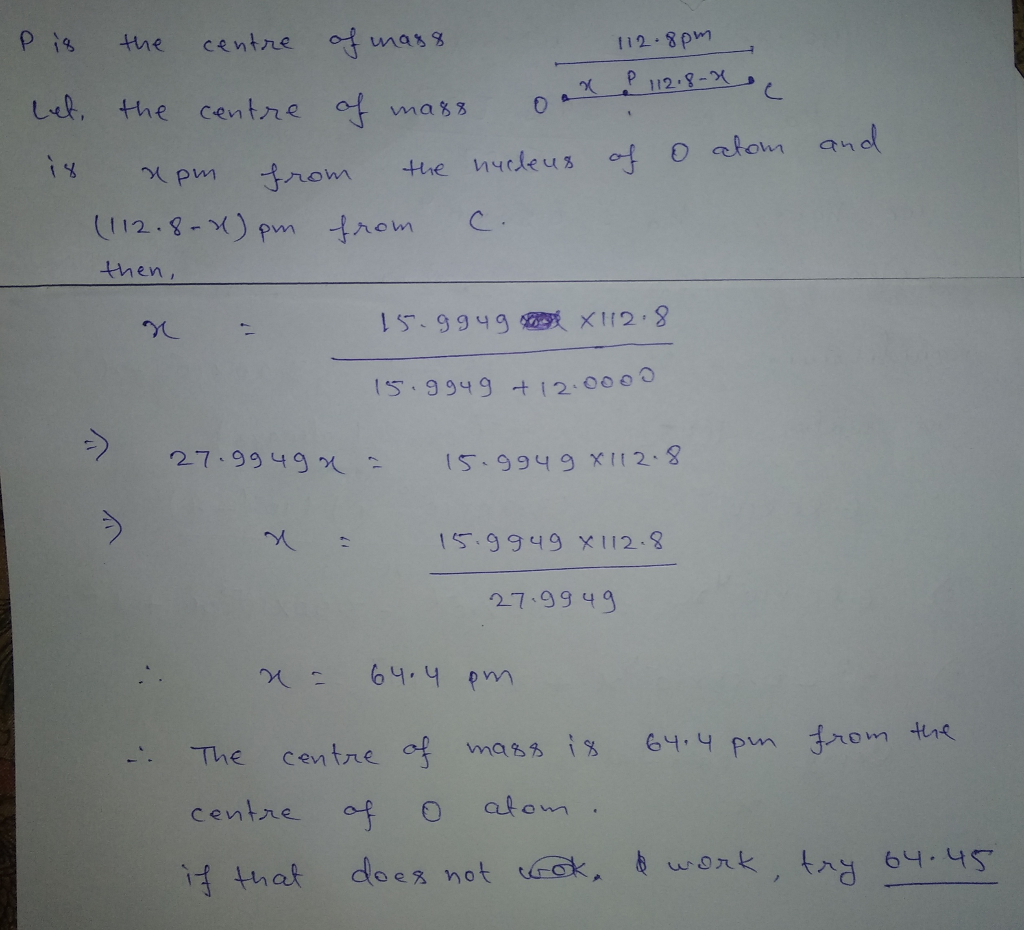Comment if any problem.

#### Earn Coins

Coins can be redeemed for fabulous gifts.

Similar Homework Help Questions
• ### O ELEC Finding the minimum uncertainty in a position or velocity m. STRUCTURE An atom of xenon ha...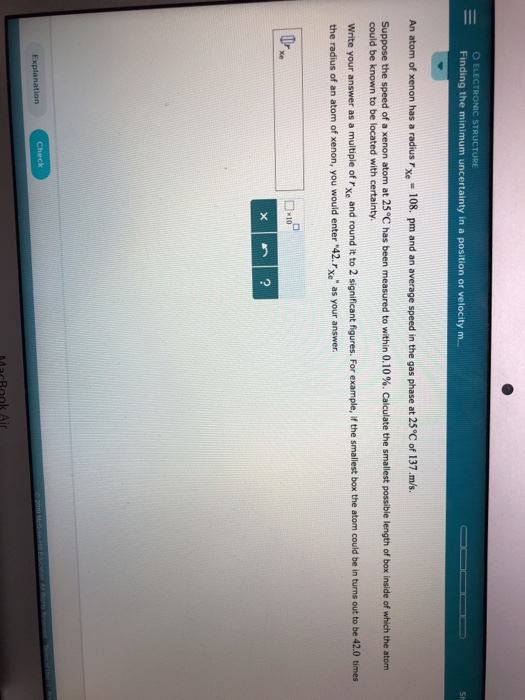O ELEC Finding the minimum uncertainty in a position or velocity m. STRUCTURE An atom of xenon has a radius rxe 108. pm and an average speed in the gas phase at 25°C of 137.m/s. Suppose the speed of a xenon atom at 25°C has been measured to within 0.10%. Calculate the smallest possible length of box inside of which the atom could be known to be located with certainty. Write your answer as a multiple of rxe and round...

• ### 2. The force constant for the CO molecule is 1860 N m-1 a. Calculate the reduced mass of CO. The ...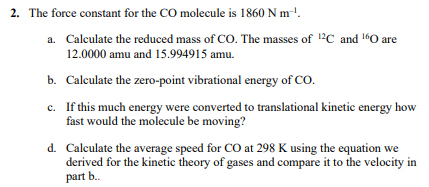2. The force constant for the CO molecule is 1860 N m-1 a. Calculate the reduced mass of CO. The ses of C and O are 12.0000 amu and 15.994915 amu. b. Calculate the zero-point vibrational energy of CO If this much energy were converted to translational kinetic energy how fast would the molecule be moving c. d. Calculate the average speed for CO at 298 K using the equation we derived for the kinetic theory of gases and compare...

• ### Problem 18.37 Part A Calculate the reduced mass for H2, which has a bond length of...

Problem 18.37 Part A Calculate the reduced mass for H2, which has a bond length of 75.69 pm . Part B Calculate the moment of inertia for H2, which has a bond length of 75.69 pm . Part C Calculate the angular momentum in the J=1 rotational level for H2, which has a bond length of 75.69 pm . Part D Calculate the energy in the J=1 rotational level for H2, which has a bond length of 75.69 pm .

• ### Could you please show me how to approach the incorporating mass into finding the center of...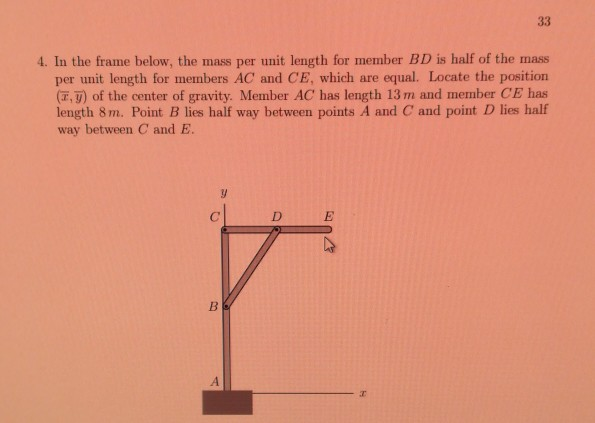Could you please show me how to approach the incorporating mass into finding the center of gravity, so far I know how to find the center, but without the mass complication. Thanks . In the frame below, the mass per unit length for member BD is half of the mass per unit length for members AC and CE, which are equal. Locate the position (T, y) of the center of gravity. Member AC' has length 13 m and member CE...

• ### Part IV: Bond Energy and Bond Length The strength of a covalent bond is measured by the bond energy. There are tabl...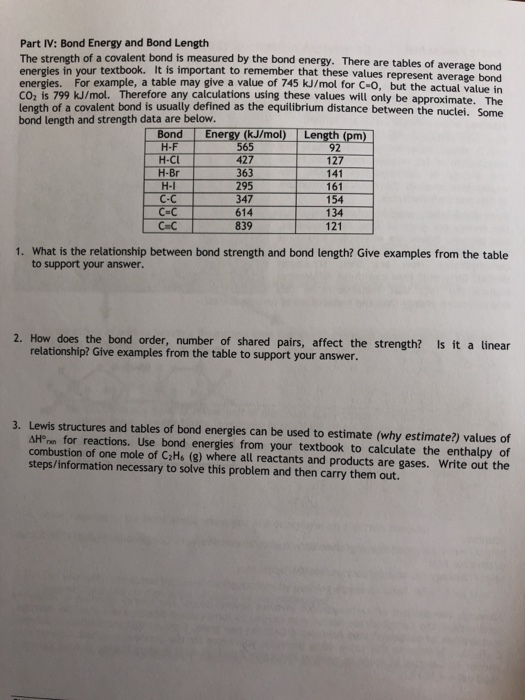Part IV: Bond Energy and Bond Length The strength of a covalent bond is measured by the bond energy. There are tables of average bond energies in your textbook. It is important to remember that these values represent average bond energies. For example, a table may give a value of 745 kJ/mol for C-O, but the actual value in Co, is 799 kJ/mol. Therefore any calculations using these values will only be approximate, The length of a covalent bond is...

• ### What is the position of the center of mass of the part? 6 point (s) m...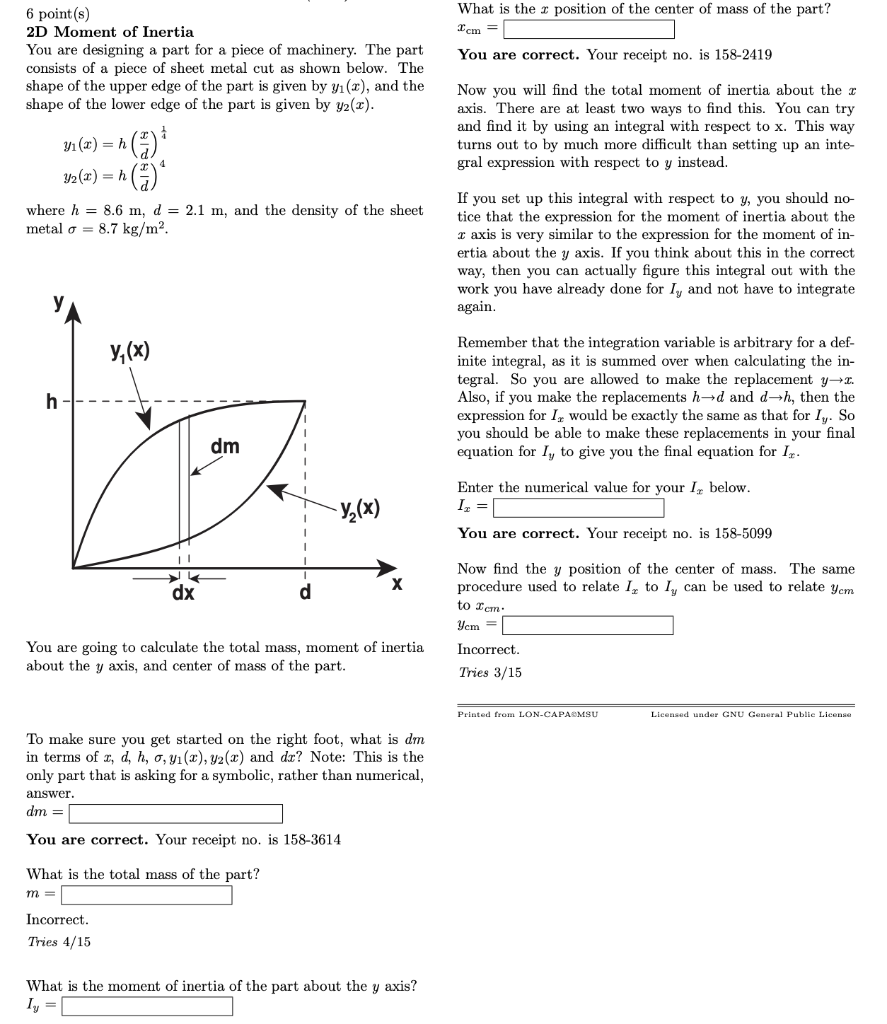What is the position of the center of mass of the part? 6 point (s) m 2D Moment of Inertia You are designing a part for a piece of machinery. The part consists of a piece of sheet metal cut as shown below. The shape of the upper edge of the part is given by y1(x), and the shape of the lower edge of the part is given by y2(x) You are correct. Your receipt no. is 158-2419 Now you...

• ### Please Answer within the hour and Show work. Thank you. No answer submission is required for...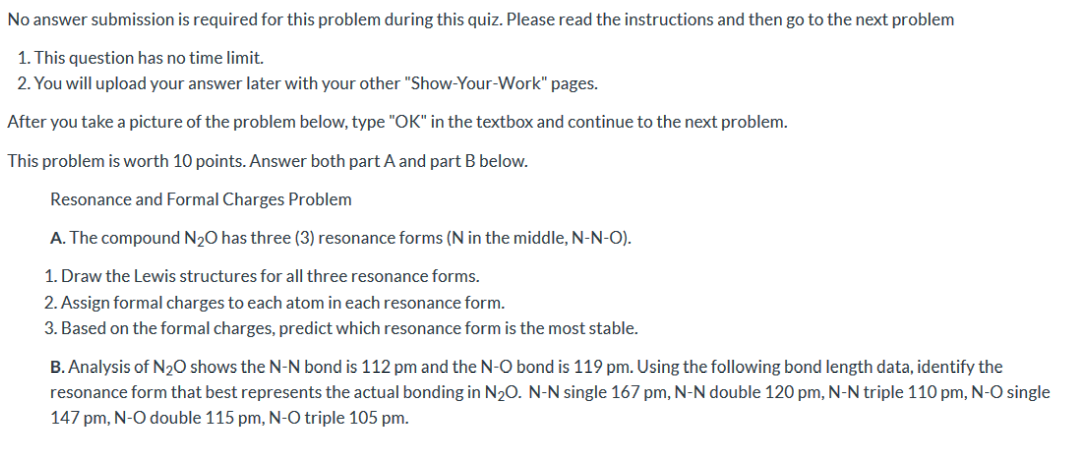Please Answer within the hour and Show work. Thank you. No answer submission is required for this problem during this quiz. Please read the instructions and then go to the next problem 1. This question has no time limit. 2. You will upload your answer later with your other "Show-Your-Work" pages. After you take a picture of the problem below, type "OK" in the textbox and continue to the next problem. This problem is worth 10 points. Answer both part...

• ### Suppose two children are using a uniform seesaw that is 3.00 m long and has its center of mass over the pivot. The first...

Suppose two children are using a uniform seesaw that is 3.00 m long and has its center of mass over the pivot. The first child has a mass of 30.0 kg and sits 1.40 m from the pivot. (a) Calculate where the second 18.0 kg child must sit to balance the seesaw. (b) What is unreasonable about the result? (c) Which premise is unreasonable, or which premises are inconsistent? Please show work and explanations.

• ### Interactive Exercises 9.04: Center of Mass (Robotic Arm) Robotic arms have been used on factory assembly...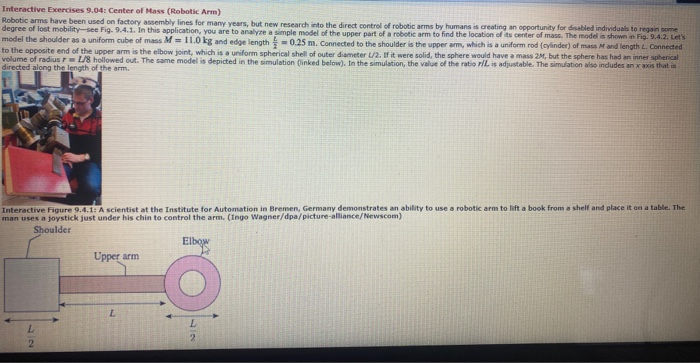Interactive Exercises 9.04: Center of Mass (Robotic Arm) Robotic arms have been used on factory assembly lines for many years, but new research into the direct control of robotic arms by humans is creating an opportunity for disabled individuals to regain some degree of lost mobility-see Fig. 9.4.1. In this application, you are to analyse a simple model of the upper part of a robotic arm to find the location of its center of mass. The model is shown in...